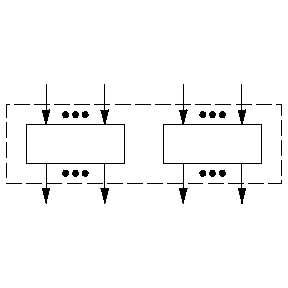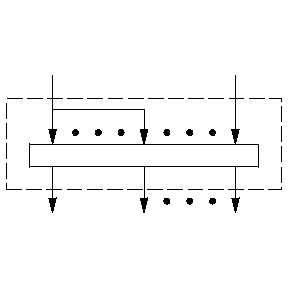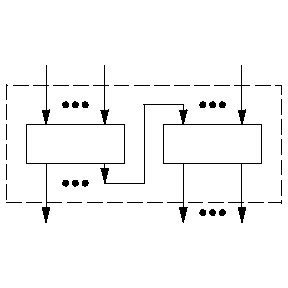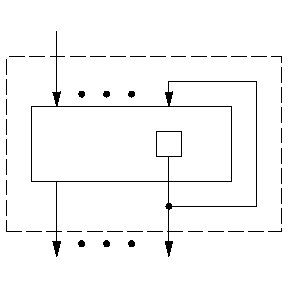# Automaton, finite

A mathematical model of a system with a finite memory which processes discrete information. Finite automata are one of the most important types of control systems (cf. Control system). Substantially, a finite automaton may be described as a system with input and output channels that is in one of $n$ states $s _ {1} \dots s _ {n}$ at any discrete moment of time. These moments constitute the time set. At each such moment signals — that is, letters from the input alphabet — are fed into the input channel and signals — that is, letters from the output alphabet — are produced by the output channel. Depending on the particular point of view, such system may include formal systems (cf. Formal system), real automata, living organisms, etc.

The concept of a finite automaton may be defined from different points of view. In the macro approach, i.e. when the only feature of interest is the external behaviour of the system, a finite automaton may be defined as a class of functions, as a finite oriented graph or (in algebraic form) as a finite algebra with unary operations (cf. Automata, methods of specification of). If the micro-approach is adopted, a finite automaton is defined as a set of elements and a scheme of their interconnections, i.e. not only the functioning of the automaton but also its structure is considered. This conception is accordingly known as structural, while the finite automata themselves are known as structural automata or automata networks.

## Macro approach.

A finite automaton is a system $(A, S, B, \phi , \psi )$ where $A, S, B$ are finite alphabets, usually non-empty, known, respectively, as the input alphabet, the set of states and the output alphabet; $\phi$ is the transition function, which maps the set $S \times A$ into $S$; $\psi$ is the output function, which maps $S \times A$ into $B$. Such finite automata are sometimes known as Mealy automata. If the output function $\psi$ maps $S$ into $B$( i.e. is independent of the letters of the input alphabet), the finite automaton is known as a Moore automaton. Any Moore automaton is also a Mealy automaton.

The most important characteristic of a finite automaton is its behaviour (cf. Automaton, behaviour of an), which may be defined in different ways. Depending on the kind of behaviour under consideration, finite automata may be grouped into transformers, acceptors (identifiers), generators, etc. In order to define the main types of behaviour of finite automata, the functions $\phi$ and $\psi$ are extended to the set $S \times A ^ {*}$( where $A ^ {*}$ is the set of all words over $A$, including the empty word $\wedge$):

$$\phi ( s, \wedge ) = s,\ \phi ( s, \alpha a ) = \phi ( \phi ( s, \alpha ), a ),$$

$$\psi ( s, \wedge ) = \wedge ,\ \psi ( s, \alpha a ) = \psi ( \phi ( s, \alpha ), a ) ,$$

where $s \in S, \alpha \in A ^ {*} , a \in A,$ while $\alpha a$ denotes the word obtained by adjoining the letter $a$ to the word $\alpha$. Thus, the extension of the functions $\phi (s, \alpha )$ and $\psi (s, \alpha )$ to arbitrary $s$ and $\alpha$ describes, respectively, the state into which the automaton passes from the state $s$ under the effect of the input word $\alpha$, and the output letter which is produced by the automaton at the moment of entry of the last letter of the input word $\alpha$. Let $\alpha] _ {n}$ denote the initial segment of length $n$ of the word $\alpha$, and let $\overline \phi \; (s, \alpha )$ and $\overline \psi \; (s, \alpha )$ be the words over $S$ and $B$ defined as follows:

$$\overline \phi \; ( s, \alpha ) = \phi ( s, \alpha] _ {1} ) \psi (s, \alpha] _ {2} ) \dots \phi ( s, \alpha ),$$

$$\overline \psi \; ( s, \alpha ) = \psi ( s, \alpha] _ {1} ) \psi (s, \alpha] _ {2} ) \dots \psi ( s, \alpha ).$$

The functions $\overline \phi \; (s, \alpha )$ and $\overline \psi \; (s, \alpha )$ describe the sequence of states assumed by the automaton during inputting the letters of the word $\alpha$, and also the output word, i.e. the sequence of letters of the output alphabet put out by the automaton when acted upon by the input word $\alpha$. The ternary relation

$$F = \{ {( \alpha , \overline \phi \; ( s, \alpha ), \overline \psi \; ( s, \alpha )) } : { \alpha \in A ^ {*} , s \in S } \}$$

is known as the functioning (operation) of the finite automaton $\mathfrak A = (A, S, B, \phi , \psi )$. The functions $\overline \phi \;$ and $\overline \psi \;$ are extended in a natural manner to infinite sequences (superwords) over $A$. For this reason, the functioning of a finite automaton is sometimes understood to mean a relation of the type $F$ in which $\alpha$ is an arbitrary superword. In such a case the value of the function $\phi (s, \alpha )$ is defined as the set of those and only those states which are encountered in the sequence $\overline \phi \; (s, \alpha )$ an infinite number of times.

A finite automaton with a designated initial state $s _ {1}$ is known as initialized and is denoted by $\mathfrak A _ {s _ {1} }$. The behaviour of an initialized finite automaton $\mathfrak A _ {s _ {1} }$ is usually defined as one of the following four relations.

1) The function $f ( \alpha ) = \overline \psi \; ( s _ {1} , \alpha )$, which maps $A ^ {*}$ into $B ^ {*}$( or $A ^ \infty$ into $B ^ \infty$, where $A ^ \infty$ and $B ^ \infty$ are the sets of all superwords over $A$ and $B$ respectively). This function is called computable or realizable by the initialized finite automaton $\mathfrak A _ {s _ {1} }$.

2) The set $L _ {S ^ { \prime } } \subseteq A ^ {*}$, which is defined for a given $S ^ { \prime } \subseteq S$ of the final states as follows:

$$L _ {S ^ { \prime } } = \{ {\alpha \in A ^ {*} } : {\phi ( s _ {1} , \alpha ) \in S ^ { \prime } } \} .$$

The set $L _ {S ^ { \prime } }$ is called an event representable by the finite automaton $\mathfrak A _ {s _ {1} }$ with set $S ^ { \prime }$ of final states.

3) The set of values of the function $f ( \alpha ) = \overline \psi \; ( s _ {1} , \alpha )$ for all possible $\alpha$ from $A ^ {*}$, called the set enumerable by the given finite automaton $\mathfrak A _ {s _ {1} }$.

4) The set $M _ \gamma \subseteq A ^ \infty$, defined for a system $\gamma$ of subsets of $S$ as follows:

$$M _ \gamma = \{ {\alpha \in A ^ \infty } : {\phi ( s _ {1} , \alpha ) \in \gamma } \} .$$

The set $M _ \gamma$ is known as a super-event, representable by the finite automaton $\mathfrak A _ {s _ {1} }$ with a system $\gamma$ of subsets of final states. Finite automata with behaviour of type 1) are known as finite transformers, while those with behaviour of type 2) are known as finite identifiers or acceptors.

If the Cartesian products $A _ {1} \times \dots \times A _ {m}$ and $B _ {1} \times \dots \times B _ {n}$ are taken as the input and output alphabets, respectively, behaviour of type 1) will be a tuple of $n$ functions of $m$ arguments. One says in such a case that the automaton has $m$ inputs and $n$ outputs, the alphabets $A _ {1} \dots A _ {m}$ and $B _ {1} \dots B _ {n}$ being called input and output alphabets of such an automaton, respectively. The class of events representable by a finite automaton and the class of functions computable by a finite automaton may be described by various mathematical tools. The principal result is that events representable by a finite automaton are identical with so-called regular events, while functions computable by a finite automaton are identical with finitely-determined functions (cf. Finitely-determined function). In addition, the class of sets enumerable by a finite automaton is identical with the class of events representable by a finite automaton.

Regarding behaviour of types 2), 3) and 4), Moore automata are equivalent to Mealy automata in the sense that to each Mealy automaton there corresponds an equivalent (i.e. displaying the same behaviour) Moore automaton, and vice versa. This is not true of behaviour of type 1). However, if in a Moore automaton functions of the form

$$\overline \psi \; ( s, \alpha ) = \psi ( \phi ( s, \alpha] _ {1} ) ) \psi ( \phi ( s, \alpha] _ {2} ) ) \dots \psi ( \phi ( s, \alpha ) ),$$

are taken instead of $\overline \psi \;$, Moore automata will be equivalent to Mealy automata.

A Moore automaton $\mathfrak A = (A, S, B, \phi , \psi )$ is said to be an autonomous automaton, or a generator, if the transition function is independent of the letters of the input alphabet. The behaviour of an initialized autonomous automaton $\mathfrak A _ {s _ {1} }$ is usually understood to mean the superword

$$\psi ( s _ {1} ) \psi ( \phi ( s _ {1} )) \psi ( \phi ^ {2} ( s _ {1} ) ) \dots ,$$

where $\phi ^ {n+1} (s) = \phi ( \phi ^ {n} (s))$. This sequence is periodic apart from a certain initial segment.

A finite automaton $\mathfrak A$ is said to be a transition system if $B = S$ and if, for any $s$ from $S$, the equation $\overline \psi \; (s, a) = s$ is true, or if the output alphabet $B$ is empty. Thus, a transition system is completely defined by the three parameters $(A, S, \phi )$.

The concepts of a finite automaton and functioning of a finite automaton may be defined by several equivalent methods (cf. Automata, methods of specification of; Automaton, behaviour of an). The so-called canonical equations are extensively employed. For any word $\alpha$ let $\alpha (t)$ denote the $t$- th letter of $\alpha$ and let $| \alpha |$ denote the length of $\alpha$. The functioning $F$ of a finite automaton $\mathfrak A$ will then comprise those and only those word triplets $( \alpha , \sigma , \beta )$ that satisfy the following conditions: 1) $| \alpha | = | \beta | = | \sigma | - 1$; 2) for each $t$ such that $1 \leq t \leq | \alpha |$ the equalities $\sigma (t + 1) = \phi ( \sigma (t), \alpha (t))$, $\beta (t) = \psi ( \sigma (t), \alpha (t))$ hold. To define the behaviour of an initialized finite automaton $\mathfrak A _ {s _ {1} }$ one must add the equality $\sigma (1) = s _ {1}$. The totality of these equalities unambiguously determines the behaviour of an initialized finite automaton, and is usually referred to as the canonical equations. Canonical equations are extensively employed in the analysis and synthesis of automata.

## Micro-approach.Figure: a014140a

Consider a set of elements consisting of a finite automaton as defined above with input and output alphabets of the form $A ^ {n}$, where $A$ is a finite alphabet, identical for all elements. The construction rules of structural finite automata from these elements describe the permitted unions (identifications) of the inputs and outputs, and thus also define the input and output sets of the finite automaton thus obtained.Figure: a014140b

The most important example of such finite automata are logical networks. One version of this concept is presented below. In this case $A = \{ 0, 1 \}$ and the elements are the so-called functional elements, which represent a finite automaton with a single state, and also certain special Moore automata, called delays. These are distinguished by the fact that they have two states, which are usually denoted by the letters 0 and 1 of the input alphabet, and that the transition and output functions satisfy the conditions $\phi (a, b) = b$ and $\psi (a) = a$. Since functional elements have only one state, they are completely determined by the output function $\psi$, which in this case is a Boolean function of $n$ arguments, where $n$ is the number of inputs of the functional elements. The elements themselves are initialized logical networks whose inputs and outputs correspond, respectively, to the inputs and outputs of the elements. Subsequent construction of more complex logical networks is performed according to the following rules.

1) The union of two logical networks is a logical network whose inputs and outputs are, respectively, those of the two logical networks (Fig. a).

2) The result of identifying two arbitrary inputs of a logical network is a logical network whose outputs are the same as those of the initial logical network and whose inputs are the same as those of the initial logical network, except for one of the identified inputs (Fig. b).

3) The result of connecting one output of a logical network to an input of another logical network is a logical network. Its inputs are all the inputs of the first logical network and those inputs of the second logical network that are not identified with the output of the first logical network; the outputs are all the outputs of both logical networks (Fig. c).Figure: a014140c

4) The result of identification of the output of a logical network that is the output of a delay element with any input of the same logical network is a logical network whose inputs are all the inputs of the initial logical network except for the identified input, and whose outputs are all the outputs of the initial logical network (Fig. d). With certain restrictions this rule may also be applied to outputs that are not outputs of a delay element of a logical network.Figure: a014140d

5) In any logical network it is possible to consider as output only some of the outputs as defined in 1–4 above. Logical networks obtained from functional elements by the rules 1, 2, 3 and 5 are usually said to be diagrams of functional elements.

The essential functioning of a logical network may be substantially described as follows. Let specified input letters be assigned to all inputs of the logical network at the moment $t$ and let the states of the delay elements be given. In this situation letters will be assigned to all inputs and outputs of the elements of the logical network, in particular to all outputs of the logical network, in accordance with the following rules. If the letters $a _ {i _ {1} } \dots a _ {i _ {n} }$ have been assigned to the inputs of a functional element with output function $\psi (x _ {1} \dots x _ {n} )$, the letter assigned to its output at this moment will be the value $\psi (a _ {i _ {1} } \dots a _ {i _ {n} } )$. If at the moment $t$ the delay is in state $a$, the letter $a$ is assigned at the same moment to the output. Identical letters are assigned to identified inputs and outputs. Moreover, at the moment $t + 1$ the states of the delay are determined by the input letters at the moment $t$, as was stated above, i.e. $\phi (a, b) = b$. Thus, if the initial states of the delay are specified, the logical network defines a certain mapping of input sequences over the alphabet $A ^ {m}$ into output sequences over the alphabet $A ^ {n}$, where $m \geq 1$ is the number of inputs and $n \geq 1$ is the number of outputs of the logical network. The class of such mappings is identical with the class of functions realized by finite automata in the first meaning of the word (i.e. with the class of finitely-determined functions), since the functioning of the logical network as described above is identical with the functioning of the finite automaton $(A ^ {m} , S, A ^ {n} , \phi , \psi )$, where $m$ is the number of inputs, $n$ is the number of outputs of the logical network and $S$ is the Cartesian product of the sets of states of all delays in the logical network; the transition function $\phi$ is a coordinate-wise application of the transition functions of the delays, while the output function $\psi$ is determined in accordance with the functioning of functional elements and delay elements as described above.

For instance, let the elements consist of delays (marked as rectangles in Fig. e) and of functional elements with output functions $\overline{x}\; , x \lor y$ and $x \& y$( marked as triangles with the respective symbols of the functions).Figure: a014140e

Fig. eshows a logical network which, at the moment of time $t$, outputs letter 1 if and only if the input letters at the moments $0 \dots t$ contain an odd number of letters "1" (at the initial moment the delay has the value 0; letters $a$ and $b$ denote, respectively, the input and the output of the logical network). If $s(t), a(t)$ and $b(t)$ denote, respectively, the state, the input letter and the output letter at the moment $t$, the functioning of such a logical network may be defined by the canonical equations:

$$s ( 0 ) = 0,\ s ( t+1 ) = s ( t ) + a ( t ) ( \mathop{\rm mod} 2 ), \ b ( t ) = s ( t ).$$

If the macro-approach is adopted, this automaton can be represented in the form $(A, S, A, \phi , \psi )$ where $A = S = \{ 0, 1 \}$, $\phi (s, a) = s + a$( $\mathop{\rm mod} 2$) and $\psi (s, a) = s$.

The concept of a finite automaton was the starting point of the modern theory of automata (cf. Automata, theory of), which is also concerned with various modifications and generalizations of this concept.

How to Cite This Entry:
Automaton, finite. Encyclopedia of Mathematics. URL: http://encyclopediaofmath.org/index.php?title=Automaton,_finite&oldid=53282
This article was adapted from an original article by V.B. KudryavtsevYu.I. Yanov (originator), which appeared in Encyclopedia of Mathematics - ISBN 1402006098. See original article